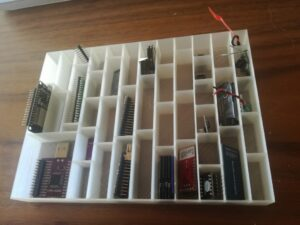I had too many micro controllers and sensors and I wanted to have simple box where I can put them and easily find my modules again.

This is the perfect use case for 3D printing: Of cause the modules and micro controllers come in different sizes so the design needed to reflect this. It is written in OpenSCAD, so you can adjust it yourself, if you want to optimize the dimensions for your specific case…Here is the source code which basically just consists of 2 loops – one for the x and the other for the y axis:

``````// some parameters
gaps = [55,50,65,30,45,50,55,40,45,25,55,33,30 ];
maxX = 205;
height = 25;
dx = 16;
gap = 1.2;

module box() {
difference() {
cube([maxX,150,height],center=false);
for (x = [1: 1 : 13]) {
for (y = [gap : gaps[x-1] : 150]) {
translate( [-dx+x*(dx+gap), y, 1.5] )
cube([dx,gaps[x-1]-gap,height], center = false);
}
}
}

translate([0,150,0]) cube([maxX,gap,height],center=false);
translate([maxX-gap,0,0]) cube([gap,150,height],center=false);
}

module boxWithBigCutout() {
box();
cube([(3*(dx+gap)+gap),58,25],center=false);
}

module bigCutout(){
translate([gap,gap,1.5]) cube([(3*(dx+gap)+gap)-(2*gap),58-(2*gap),25],center=false);
}

module boxAlt() {
difference() {
boxWithBigCutout();
bigCutout();
}
}

boxAlt();
//box();
``````